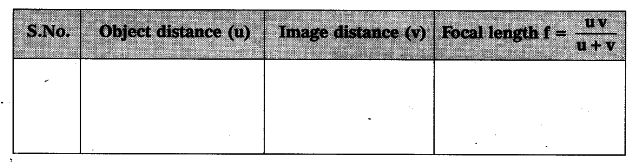# How do you find the focal length of a lens experimentally?

Aim : To determine the focal length of a convex lens.
Apparatus : Convex lens, meter scales, V-stand, screen, candle.
Procedure :
i) Place the convex lens on a ‘V’ stand. .
ii) Light a candle and take it far away from the lens along the principal axis.
iii) Adjust the screen on the other side of the lens to get a clear image on it.
iv) Measure the distance between the ‘V’ stand and the screen which gives a rough idea of focal length of the lens.
U-V Method : (Displacement method)
Now place the candle at a distance of 60 cm from the lens, such that the flame of the candle lies along the principal axis of the lens.
Adjust the screen t© get a clear image and measure the values of object distance (u) and image distance (v) and note them in a table.
Repeat the above step for various distances like 50 cm, 40 cm, 30 cm etc., note the corresponding (v) values and note them in the table.Calculate the focal length for each case, you find that the focal length remains constant for a given lens.